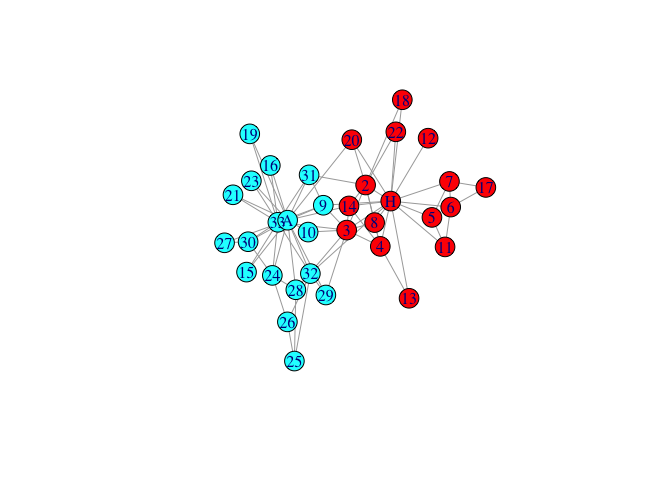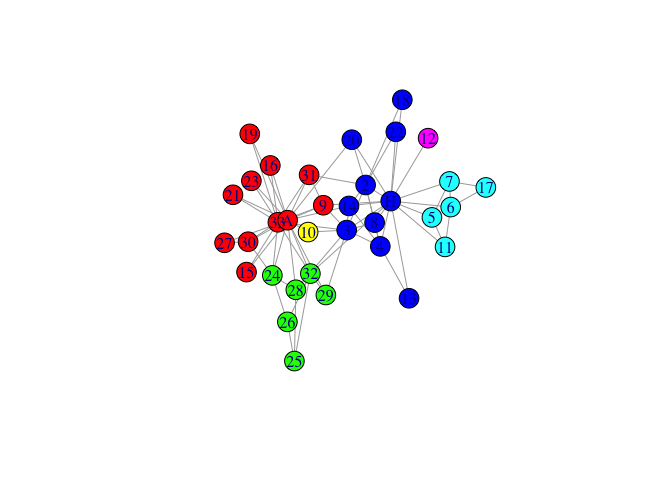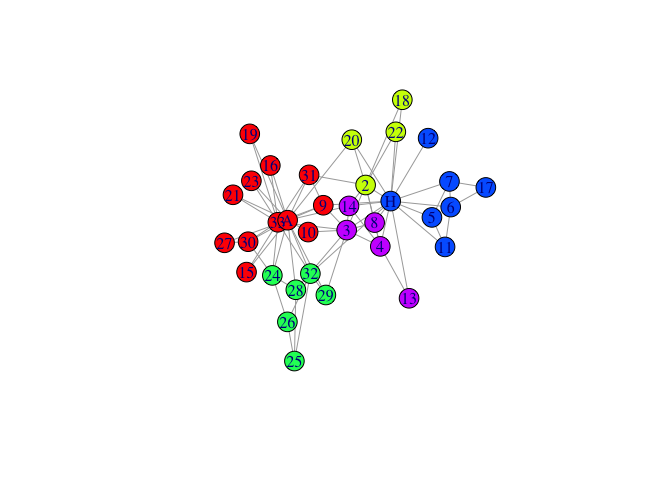# rSpectral

The goal of ‘rSpectral’ is to make Spectral Modularity graph clustering method available to most of R graph frameworks.

## Installation

You can install the development version of rSpectral from GitHub with:

``````# install.packages("devtools")
devtools::install_github("cmclean5/rSpectral")``````

## Example

This is a basic example which shows you how to solve a common problem

1. load the karate club graph and plot Faction membership:
``````library(rSpectral)
library(igraph)
#>
#> Attaching package: 'igraph'
#> The following objects are masked from 'package:stats':
#>
#>     decompose, spectrum
#> The following object is masked from 'package:base':
#>
#>     union
data(karate, package="igraphdata")
l<-layout_nicely(karate)
memT<-V(karate)\$Faction
palette <- rainbow(max(as.numeric(memT)))
plot(karate,vertex.color=palette[memT],layout=l)``````1. run spectral clustering on graph
``mem0<-igraph::membership(rSpectral::spectral_igraph_communities(karate))``
1. plot the graph with membership colors
``````palette <- rainbow(max(as.numeric(mem0)))
plot(karate,vertex.color=palette[mem0],layout=l)``````1. run spectral clustering on graph, fixing neighbouring nodes found in same community
``````mem1<-igraph::membership(
rSpectral::spectral_igraph_communities(karate, fix_neig=1))``````
1. and plot again
``````palette <- rainbow(max(as.numeric(mem1)))
plot(karate,vertex.color=palette[mem1],layout=l)``````1. run spectral clustering - fixing neighbouring nodes, Cnmin=5
``````mem1.5<-igraph::membership(
rSpectral::spectral_igraph_communities(karate, fix_neig=1,Cn_min=5))``````
1. and the last plot
``````palette <- rainbow(max(as.numeric(mem1.5)))
plot(karate,vertex.color=palette[mem1.5],layout=l)```````GraphNEL` objects could be processed similarily, all other graph types could be converted either to `igraph` or to `GraphNEL` by packages such as `Intergraph`.